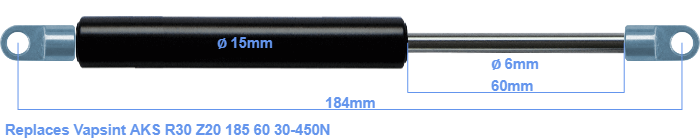# Replacement for Vapsint AKS R30 Z20 185 60 30-450N

\$28.37

Replacement gas spring for the Vapsint AKS R30 Z20 185 60 30-450 Newton. The eye has a thickness of 3mm and a hole diameter of 6.1mm. Brand: Hahn.
 Force Choose an option30 Newton40 Newton50 Newton60 Newton80 Newton100 Newton120 Newton140 Newton150 Newton160 Newton180 Newton200 Newton220 Newton240 Newton250 Newton260 Newton280 Newton300 Newton320 Newton340 Newton350 Newton360 Newton380 Newton400 Newton420 Newton440 Newton450 NewtonClear
This gas spring is also known as Brimotech 10220002.This gas spring has a cylinder diameter of 15 mm. The diameter of the rod totals 6 mm. The stroke of the gas spring is 60 millimeter. The total length of this replacement gas spring is 184 mm. Note: this is the total length between the rotation points of the two mounting parts. Without the mounting parts this gas spring is 152 mm long (thread to thread). The force of this replacement is 30-450 Newton. This is not an original gas spring from Vapsint, but a so called replacement gas spring. This replacement gas spring is a Stabilus Industry Line gas spring. The force (30-450 N) and dimensions are identical to the original.